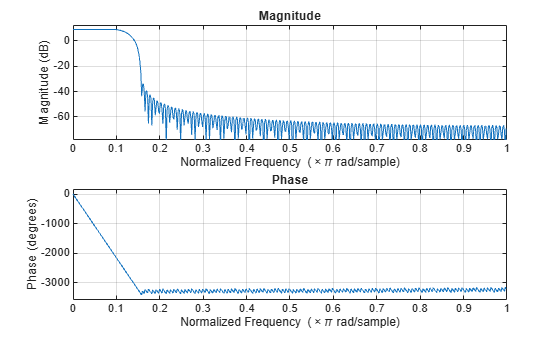# freqz

Frequency response of discrete-time filter

## Syntax

``````[filtresp,w] = freqz(rcfilter)``````
``````[filtresp,w] = freqz(rcfilter,n)``````
``````[filtresp,w] = freqz(___,'Arithmetic',arithType)``````
``freqz(rcfilter)``

## Description

``````[filtresp,w] = freqz(rcfilter)``` returns `filtresp`, the complex frequency response of the specified filter. The output `w` contains the frequencies (in radians per sample) at which the function evaluates the frequency response.```
``````[filtresp,w] = freqz(rcfilter,n)``` returns the complex frequency response of the specified filter and the corresponding frequencies at `n` points that are equally spaced around the upper-half of the unit circle (from 0 to π).This function uses the transfer function that is associated with the specified filter to calculate the frequency response of the filter with the current coefficient values.```
``````[filtresp,w] = freqz(___,'Arithmetic',arithType)``` specifies the type of arithmetic that the function uses to evaluate the filter response. You can use any input combination from the previous syntaxes.```

example

````freqz(rcfilter)` plots the magnitude and unwrapped phase of the frequency response of the specified filter by using the `fvtool` function.For more input options, see the Signal Processing Toolbox™ `freqz` function.```

## Examples

collapse all

Evaluate the frequency response of an RRC filter.

Create a transmit RRC filter System object™. Evaluate the frequency response by using the `freqz` object function.

```rrcFilt = comm.RaisedCosineTransmitFilter( ... "FilterSpanInSymbols",30, ... "RolloffFactor",0.25); freqz(rrcFilt)```## Input Arguments

collapse all

Filter, specified as one of these System objects.

Number of points over which the frequency response is computed, specified as positive integer. For faster computations (performed using FFTs), specify `n` as a power of two.

Data Types: `double`

Arithmetic used in the filter analysis, specified as `'double'`, `'single'`, or `'Fixed'`. When you do not specify the arithmetic type and the filter System object is unlocked, the analysis tool assumes a double-precision filter. When you do not specify the arithmetic type and the System object is locked, the function performs the analysis based on the data type of the locked input.

The `'Fixed'` value applies to filter System objects with fixed-point properties only.

When you specify this input as `'Fixed'` and the filter object has the data type of the coefficients set to `'Same word length as input'`, the arithmetic analysis performed depends on whether the System object is unlocked or locked.

• If the System object is unlocked, the analysis object function cannot determine the data type of the coefficients. The function assumes that the data type of the coefficients is signed, has a 16-bit word length, and is autoscaled. The function performs fixed-point analysis based on this assumption.

• If the System object is locked –– When the input data type is `'double'` or `'single'`, the analysis object function cannot determine the data type of the coefficients. The function assumes that the data type of the coefficients is signed, has a 16-bit word length, and is autoscaled. The function performs fixed-point analysis based on this assumption.

To check if the System object is locked or unlocked, use the `isLocked` function.

When you specify this input as `'Fixed'` and the filter object has the data type of the coefficients set to a custom numeric type, the object function performs fixed-point analysis based on the custom numeric data type.

Data Types: `char` | `string`

## Output Arguments

collapse all

Frequency response, returned as a complex-valued column vector of length `n`. The function evaluates the frequency response at `n` points that are equally spaced around the upper-half of the unit circle (from 0 to π).

Data Types: `double`
Complex Number Support: Yes

Frequencies used for frequency response evaluation, returned as a column vector of length `n`. Unit are in radians per sample. The frequencies are equally spaced around the upper-half of the unit circle (from 0 to π).

Data Types: `double`

## Tips

• Several ways exist for analyzing the frequency response of filters. The `freqz` function accounts for quantization effects in the filter coefficients but does not account for quantization effects in filtering arithmetic. To account for the quantization effects in filtering arithmetic, see the `noisepsd` function.

• For faster computations (performed using FFTs), specify `n`, the number of points over which the function computes the frequency response, as a power of two.

## Algorithms

The `freqz` function calculates the frequency response for a filter from the filter transfer function Hq(z). The complex-valued frequency response is calculated by evaluating Hq(ejω) at discrete values of `w`. The input argument `n` specifies the number of equally-spaced points around the upper-half of the unit circle at which the function evaluates the frequency response.

• When you do not specify scalar sampling frequency `fs` as an input argument, the frequency ranges from 0 to π radians per sample.

• When you specify scalar sampling frequency `fs` as an input argument to `freqz`, the frequency ranges from 0 to `fs`/2 Hz. For more information about `fs`, see the Signal Processing Toolbox `freqz` function.

Introduced in R2013b